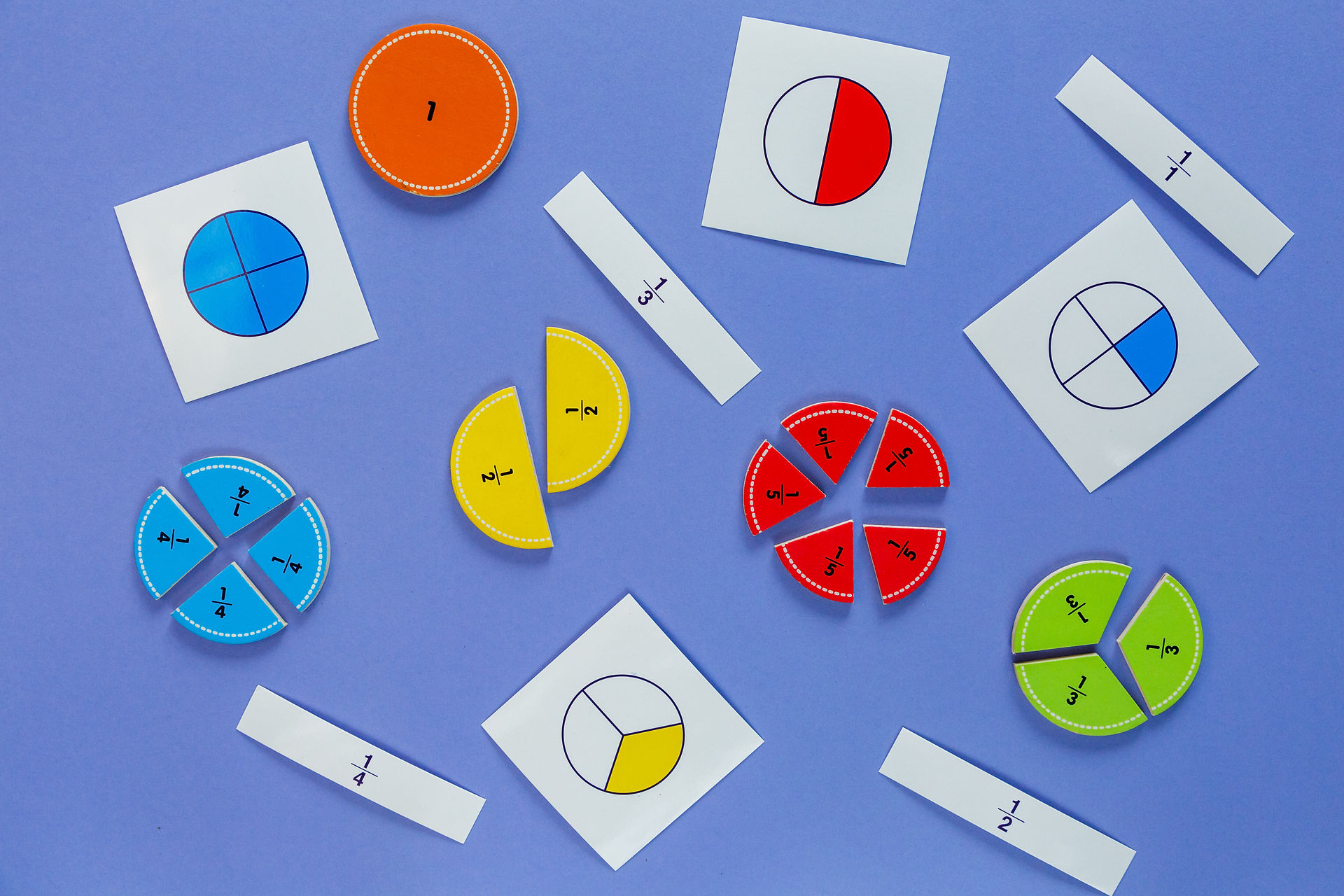top of page### Math Warm-Ups

Splat Math (by Steve Wyborney)

Fraction Talks.com

Clothesline Math (Estimation 180)

Estimation 180

### Online Games

Equivalent Fractions

Fractions on the Number Line, Equivalent Fractions, Partitioning Rectangles

Splash Math Fraction Games

A collection of games that includes many 4th grade fraction standards.

Fraction Concentration

Match fractions to an area or group model.

The Fraction Game

Decomposing fractions, equivalent fractions, combining fractions

Desmos: The Fraction Challenge

Pick a Path

A fun/challenging game with fraction operations.

Fraction (and Decimal) Models

Connecting fractions, percents, and decimals through visual modeling.

Desmos:  The Decimal Challenge

### Home Material Games

Fractions

Cover Up Fraction Game

Cùbrelo (Spanish)

Explore the relative size of fraction pieces and relationships between them.

Uncover Fraction Game

Descubrelo/Destapalo (Spanish)

Get really good at strategically exchanging fractions for equivalent fractions.

Greatest Fraction Wins!

La Fracción más Grande Gana! (Spanish)

Roll and place each digit as you explore the role of the numerator & denominator  in the value of a fraction as well as model and compare fractions. For 4th and 5th grades, play the Greatest Sum or Greatest Difference wins!

Fraction Capture

Captura la Fracción (Spanish)

This game is like the card game “War.”  Compare fractions, justify your thinking, win cards!

Fraction War (from Fractiontalks.com)

This game is worth a try!  Geometric fraction reasoning.

Fraction War Video

Fraction War Cards

Fractions In-Between Game

Fracciones en el Medio (Spanish)

Compare fractions, order fractions, justify your thinking

Target 1

En el Blanco 1 (Spanish)

Players choose cards to make 2 decimal numbers that have a sum as close to 1 as possible.

Target 2

En el Blanco 2 (Spanish)

Smallest Product Wins (Fractions)

El Producto Menor Gana!(Fracciones) (Spanish)

Roll the die and place the digit into a whole number by a fraction multiplication frame.  Modify by multiplying a fraction by a fraction.

Estimation180 Scavenger Hunt

Students measure estimate and measure their own height to nearest 1/4 inch, then find and measure objects equal to, shorter than, and taller than their own height.  Finally, they build a number line with their measurements, emphasizing spacing and placing accurately.

Decimals

The Place Value Game

Juego de Valor Posicional (Spanish)

Place Value Game Demo Video

Virtual Spinner

Decimal Place Value

Decimal Compare

Comparar con Decimales (Spanish)

Compare decimals - like the card game War.

Digital Tool:  Modeling Decimals

Decimal Double Compare

Comparación Decimal Doble (Spanish)

Digital Tool:  Modeling Decimals

Smaller to Larger - Decimals

Decimales de Menor a Mayor (Spanish)

Digital Tool:  Modeling Decimals

Decimal Maze

Laberinto Decimal- Tablero de Juego (Spanish)

Making sense of decimal operations.  What is the highest score you can get?  Use a calculator and have fun!

Decimal Maze Video

Decimal Maze on Desmos - Teacher

Decimal Maze on Desmos - Students

Other Decimal Games

### Practice

Practice Book (Fractions Units 4 & 7)

Temporarily Free from The Math Learning Center

Comparing and Ordering Decimals (Gizmos)

Online practice with interactive modeling, practice items, and answers.

Desmos:  Equivalent Fractions

Desmos: The Fraction Challenge

The Kool-Aid Kid

Fraction Estimation